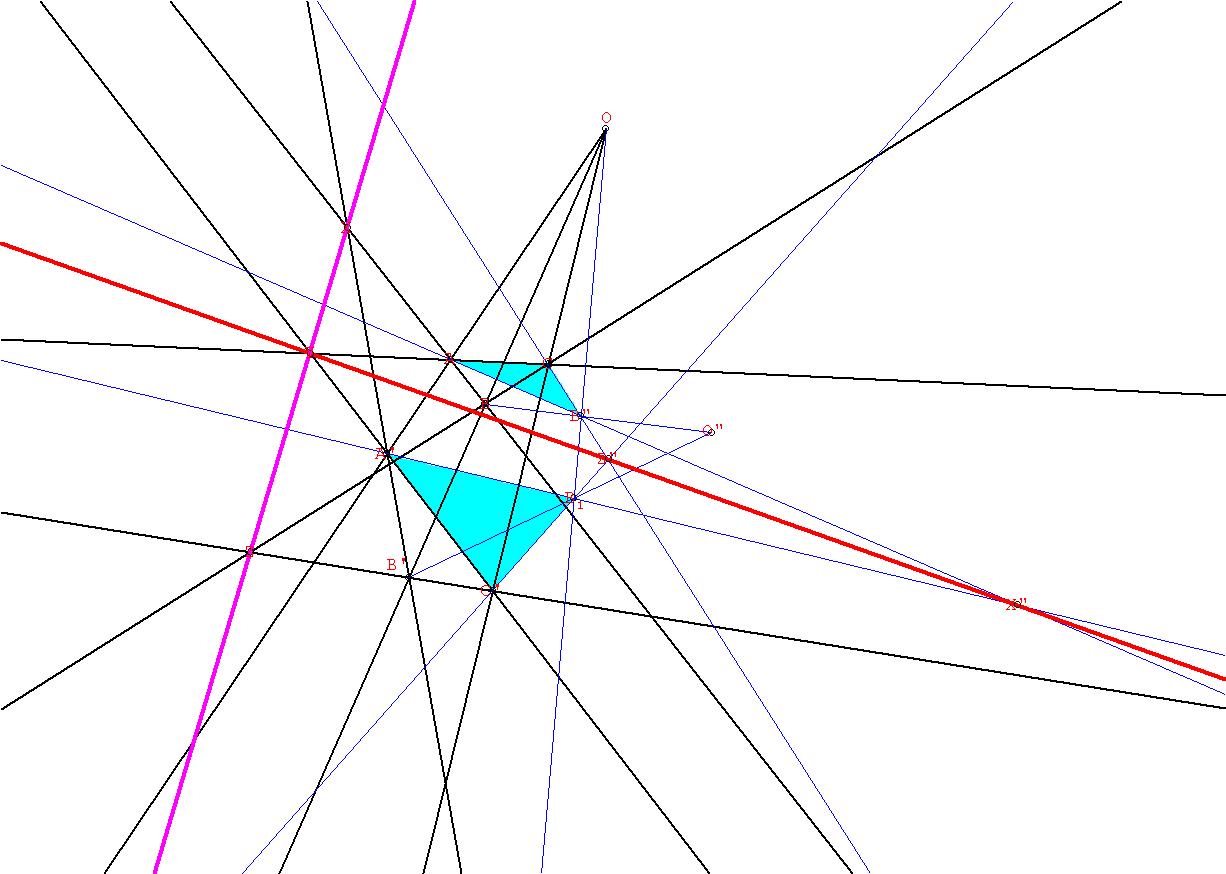Desargues' Theorem
Notes by M. Flashman
Based on proof found in Hilbert&Cohn-Vossen's Geometry and The Imagination.

• Desargues' Theorem: (in projective 3 space). If two non co-planar triangles ABC and A'B'C' are perspectively related by the center O, then the points of intersection X=AB#A'B'; Y=AC#A'C' ; and Z=BC#B'C' all lie on the same line.[# is used here to indicate the intersection of the lines.]
• Proof: The intersection of the planes determined by the two triangles is a line. This line has the points X, Y, and Z on it, because these points must lie on the intersection of the two planes.

• In a "projective spatial geometry" any pair of distinct planes would intersect in a line.  EOP.
• Notice that if we use a central projection of the spatial configuration of lines and vertices from the spatial Desargues' Theorem we have a planar configuration of lines and vertices, satisfying the same hypotheses and illustrating the same conclusion. This basic idea of projecting a result from 3-space onto the plane is used in the proof of the planar version of Desargues' Theorem as provided in the book Geometry and The Imagination by Hilbert and Cohn-Vossen.
• Desargues' Theorem: (in the (projective) plane). If two coplanar triangles ABC and A'B'C' are perspectively related by the center O, then the points of intersection X=AB*A'B'; Y=AC*A'C' ; and Z=BC*B'C' all lie on the same line.• Proof: Choose O* not in the plane ABC. Choose B* a point on BO*. Let B'* denote the intersection of O*B' with OB* in the plane OBO*.
Now triangles AB*C and A'B'* C' are perspectively related  in space by the center O.
So by Desargues' Theorem in space, the points X*=AB*#A'B'*; Y*=AC#A'C' ; and Z*=B*C#B'*C' all lie on the same line l* in space which does not pass through the point O*.
Now the plane determined by l* and O* meets the plane ABC on a line l.
Consider that O*X* #ABC = {X}, Y* = Y and O*Z* #ABC = {Z}.   Thus the three points X, Y and Z all lie on the line l.   EOP.